## General Question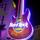# Which one of these is right (math)

Asked by Disc2021 (4491) April 22nd, 2010

3x + 5y = 30… Is it :

3x + 5y = 30
x + 5y = 30/3, x + 5y = 10
x + y = 10/5, x + y = 2
y = 2 – x

Or

3x + 5y = 30
3/5x + y = 30/5 , 3/5x + y = 6
y = 6 – 3/5x

Someone throw me a bone here…

Observing members: 0Composing members: 0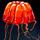When dividing by a number (such as the 3x-to-x or 5y-to-y) you need to divide all the terms that are on each of the sides.

davidgro (609)“Great Answer” (2) Flag as…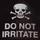I’m embarrassed to see how much algebra I’ve forgotten in the past few years. I believe you need to isolate the x and y so that one is on each side of the equals sign. Then, like @davidgro said, you have to divide all terms on each of the sides.

This is how I would solve it..

3x+5y=30
First subtract 5y to both sides
3x=30–5y
Then Divide both sides by 3
x=10–5y/3

MissAnthrope (21491)“Great Answer” (0) Flag as…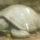Its the second one. Try this.

x = 5, y = 3

3 * 5 + 5 * 3 = 30 <—- Check! Yes, this is correct.

Or

3/5 * 5 + 5/5 * 3 = 6. <——Check! Yes, this is correct.

Here’s another way to think about it. The first statement:

3 * x + 5 * y = 30

I’m saying, “If I take 3 x’s and 5 y’s, I’ll get 30.”

If I take half as much of everything, I should get half as much of the total. So, I could say, “if I take 3/2 x’s and 5/2 y’s I’ll get 15.” Or, in math notation:

3/2 * x + 5/2 * y = 15.

roundsquare (5517)“Great Answer” (3) Flag as…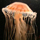paintingtheocean (51)“Great Answer” (1) Flag as…Thanks guys! Perhaps I bumped my head or something but I couldn’t figure out what seemed more “right” to me.

Disc2021 (4491)“Great Answer” (1) Flag as…Ugh.. is mine really wrong? I’m kinda mad, I used to be pretty good at this. :\

MissAnthrope (21491)“Great Answer” (1) Flag as…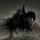Second is right. No doubt…

9doomedtodie (3113)“Great Answer” (1) Flag as…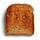@MissAnthrope I’m a Calculus student, and I’m pretty sure #1 is correct. Unless my academic career has failed me.

ShiningToast (2106)“Great Answer” (0) Flag as…Don’t think that makes you wrong @MissAnthrope , isn’t that what’s being done in the second solution?

Disc2021 (4491)“Great Answer” (0) Flag as…I probably shouldn’t chime in on math questions when I’m tired.. yeah, I see now he solved for y.

MissAnthrope (21491)“Great Answer” (1) Flag as…Same here.

ShiningToast (2106)“Great Answer” (0) Flag as…@Disc2021 :Now you can cross check the equation. Put the values of either x or y in the same equation you will get the answer 30.

y = 6 – 3/5x & x=10–5y/3.

e.g.

3(10–5y/3) + 5y = 30

30 – 5y + 5y =30

9doomedtodie (3113)“Great Answer” (2) Flag as…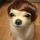The first set of working is wrong – when you divide 3 in line 2, you have to divide 3 for the whole line of eqn, i.e. x + (5/3)y = 10

The second set of working is right.

One easy way to handle this type of problems is:
1). determine in terms of which variable you want your answer to be (in your case: x or y)

2). make the other variable the subject of the equation by PUTTING EVERYTHING ELSE to the right side of the equal sign (if you want to make ‘y’ to be part of your answer, then ‘x’ will be the subject of the equation)

3x + 5y = 30 -> choose ‘x’ to be the subject of the equation
3x = 30 – 5y -> put everything else that doesn’t have the ‘x’ term to the right side of eqn
x = 10 – (5/3) y

Hope it helps! :)

ilovechoc (142)“Great Answer” (0) Flag as…Yep, I did some calculations and I was completely wrong. Woooooooooooow.

ShiningToast (2106)“Great Answer” (1) Flag as…@ShiningToast Story of my life when it comes to math. No worries.

Disc2021 (4491)“Great Answer” (0) Flag as…@Disc2021 I’m going to bed haha.

ShiningToast (2106)“Great Answer” (0) Flag as…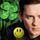The second one is right.

In the first set, you forgot to divide the 5Y term by 3 in the very first step.

LuckyGuy (38684)“Great Answer” (1) Flag as…1) Isolate the variable then do the division to get “y = ....”

2) Divide to get “y = ....” and then isolate the variable.

Solve for y instead of x and you’ll get the right answer.

roundsquare (5517)“Great Answer” (1) Flag as…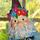@Disc2021

3x + 5y = 30… Is it :

3x + 5y = 30
x + 5y = 30/3, x + 5y = 10 <— Here, you did not divide 5y by 3. It should be 5y/3.
...............................................You need to divide all terms by 3 to be consistent.
x + y = 10/5, x + y = 2
y = 2 – x

Or

3x + 5y = 30
3/5x + y = 30/5 , 3/5x + y = 6 <— Here you divided all terms by 5. This is correct.
y = 6 – 3/5x <— Correct.

In the first one you did, if you divide all terms by 3 (instead of 5, like in the second one you did) you should get the same answer that you got in the second one you did. Both would be correct approaches.

lilikoi (10084)“Great Answer” (1) Flag as…Yay, I am so glad that I haven’t entirely forgotten what I learned!

MissAnthrope (21491)“Great Answer” (0) Flag as…or